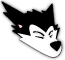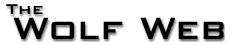User not logged in - login - register
 Home Calendar Books School Tool Photo Gallery Message Boards Users Statistics Advertise Site Info
go to bottom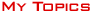|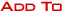|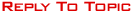Message Boards » » Trigonometry problem Page 
0EPII1
All American
41348 Posts
user info
edit post

Came across this on a website which has challenging questions for HS students. How to solve this system of equations:

sinx + siny = 1.4
cosx + cosy = 1.2

I know it can't be that difficult because it is a high school level problem and doesnt involve calc in the solution, but haven't done trig for a while, and so can't seem to solve it.

I tried 2 different approaches and got stuck on both:

Method 1:
siny = ....
cosy = ....

Square both sides of each equation, and add them together, expand the squares, and simplify to get:
2.8sinx + 2.4cosx = 3.4

How to solve that?

Method 2:
Solve both equations for y (or for x), and then equate:

arcsin(1.4 - sinx) = arccos(1.2 - cosx)

And then what?! I tried taking sin (or cos) of both sides, but then don't know how to proceed further.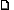1/21/2014 3:04:23 PM

Smath74
All American
93141 Posts
user info
edit post

 Quote : "Post new threads in the right section - Save us the trouble of having to move your topic to the correct section. Post in the right section to begin with. Additionally, if you post the same topic in multiple sections or post a dummy topic pointing to a topic in another section, this is grounds for immediate suspension. Nothing pisses us off more than seeing "I posted this in but no one looks there so now I am posting it in to get more attention"."1/21/2014 3:38:20 PM

0EPII1
All American
41348 Posts
user info
edit post

This is the correct section for it, I made a mistake posting it in study hall. This is "fun stuff" for me. It is *not* a test or a homework or related stuff. It is like posting a puzzle. I have asked for the other thread to be deleted.1/21/2014 3:44:28 PM

Stryver
Veteran
313 Posts
user info
edit post

There are a set of sum/difference relationships that are useful. This one should do the trick:

(sinX + sinY)/(cosX+cosY) = tan(1/2*(X+Y))

The numeric values can be substituted like so:

1.4/1.2 = tan(1/2(X+Y))

Which suggests 1/2(X+Y) = 49.4 degrees and X = 99 degrees - Y

This can be substituted back into the original equation, which I will finish later.1/21/2014 5:28:45 PM

Stryver
Veteran
313 Posts
user info
edit post

Hmmm... It didn't make a quick solution. I'll take a hack later.1/21/2014 5:46:57 PM

0EPII1
All American
41348 Posts
user info
edit post

Hey thanks for attempting.

The website gave a tip on how to proceed, and that's what I have done in method 2. But I don't know what to do after that. Actually the website said the question is a past IB Math exam question, and the question actually said to solve both equations for y and equate for solving. So perhaps that's the easiest/quickest way to solve.1/21/2014 6:15:01 PM

Stryver
Veteran
313 Posts
user info
edit post

I followed the arcsin(1.4-sinx) = arccos(1.2-sinx) method for a ways. I took the sin of both sides, canceling the arcsin and opening up that paren. Moved the 1.4 over, took the arcsin of both sides, giving
-x = arccos(1.2 - sinx) - arcsin(1.4)

From here, similar manuevers for the other side result in:
sin(x) - cos(x) = 1.2 - cos(arcsin(1.4))

This is numerically solveable (I used Wolframalpha, my other method would have been graphing) but I don't see an easy analytic solution for sin(x) - cos(x)1/21/2014 8:22:25 PM

lewisje
All American
9195 Posts
user info
edit post

It relies on the circular identity cos^2(x)+sin^2(x)=1 and the sum-to-product and product-to-sum identities, like cos(a+b)=cos(a)cos(b)-sin(a)sin(b) and cos(a)+cos(b)=2cos((a+b)/2)cos((a-b)/2):

`sin(x)+sin(y)=7/5cos(x)+cos(y)=6/5`
Square both sides of each equation:
`sin^2(x)+2sin(x)sin(y)+sin^2(y)=49/25cos^2(x)+2cos(x)cos(y)+cos^2(y)=36/25`
Then form a new system by adding and subtracting the pairs of equations:
`cos^2(x)+sin^2(x)+2cos(x)cos(y)+2sin(x)sin(y)+cos^2(y)+sin^2(y)=85/25cos^2(x)-sin^2(x)+2cos(x)cos(y)-2sin(x)sin(y)+cos^2(y)-sin^2(y)=-13/25`
Simplify:
`1+2cos(x-y)+1=17/5cos(2x)+2cos(x+y)+cos(2y)=-13/25`
Further:
`cos(x-y)=7/102cos(x+y)+2cos(x+y)cos(x-y)=-13/25`
Now substitute:
`cos(x+y)+cos(x+y)*7/10=-13/50, so (17/10)cos(x+y)=-13/50, so cos(x+y)=-13/85`
From this we can set up systems of linear equations, here assuming a canonical mapping of the half-open interval (-pi,pi] to the unit circle...
`x-y=±arccos(7/10)x+y=±(pi-arccos(13/85))`
If both are +, then x=(pi-arccos(13/85)+arccos(7/10))/2≈1.259869≈72.1852° and y=(pi-arccos(13/85)-arccos(7/10))/2≈0.4644706≈26.6122°

The other cases are left as exercises for the reader, and I'll just say that Stryver was on the right track but should have kept exact values in until the end of computation.

[Edited on January 22, 2014 at 12:04 PM. Reason : "canonical mapping" and "half-open" are fancy language for things HS students know intuitively1/22/2014 11:59:42 AM

StillFuchsia
All American
18941 Posts
user info
edit post

http://www.wolframalpha.com/input/?i=sinx+%2B+siny+%3D+1.4%2C+cosx+%2B+cosy+%3D+1.2

if you want to see it graphed as well1/22/2014 3:12:40 PM

Message Boards » Study Hall » Trigonometry problem Page 
go to top||Admin Options : move topic | lock topic